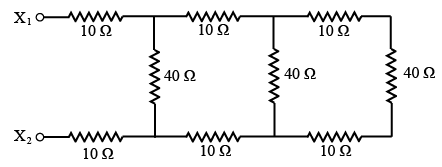# ISRO Scientist or Engineer Electronics 2006

Instructions

For the following questions answer them individually

Question 61

# Solution of $$(D^2 + 4)y = \sin^2 x$$, isQuestion 62

# The value of k for which the lines $$2x + y - 1 = 0, 4x + 3y - 3 = 0$$ and $$3x + ky - 2 = 0$$, are concurrent isQuestion 63

# A box contains 5 black and 5 red balls. Two balls are-randomly picked one after another from the box, without replacement. The probability for both balls being red isQuestion 64

# $$x^3 + x \sin x$$ isQuestion 65

# $$\int \frac{dx}{x \sqrt{x^2 - a^2}}$$ isQuestion 66

# Eigen values of $$\begin{bmatrix}-5 & 2 \\2 & -2 \end{bmatrix}$$ areQuestion 67

# The approximate equivalent resistance at the points $$X_1$$ and $$X_2$$ in the circuit shown belowQuestion 68

# An inductor supplied with 50 V ac with a frequency of 10 kHz passes a current of 7.96 mA. The value of inductor isQuestion 69

# Ina capacitor, the electric charge is stored inQuestion 70

# Oscillator requiresOR